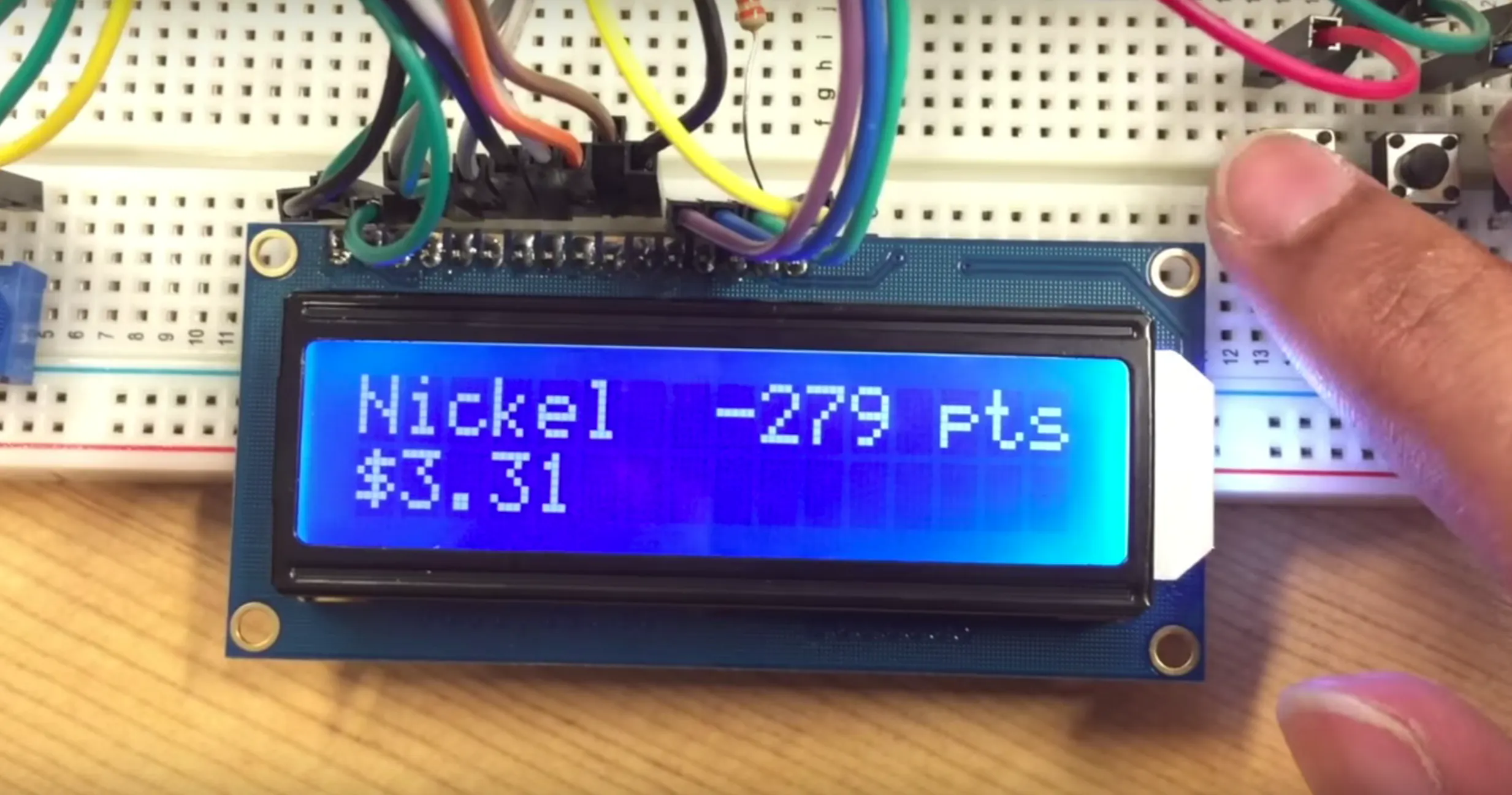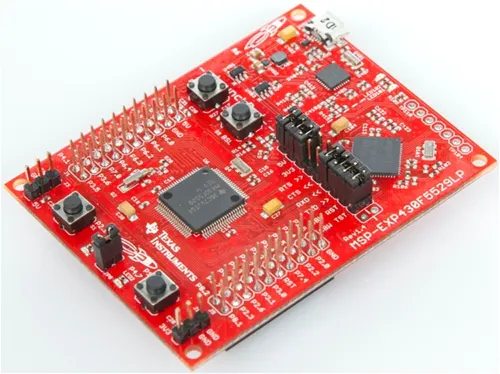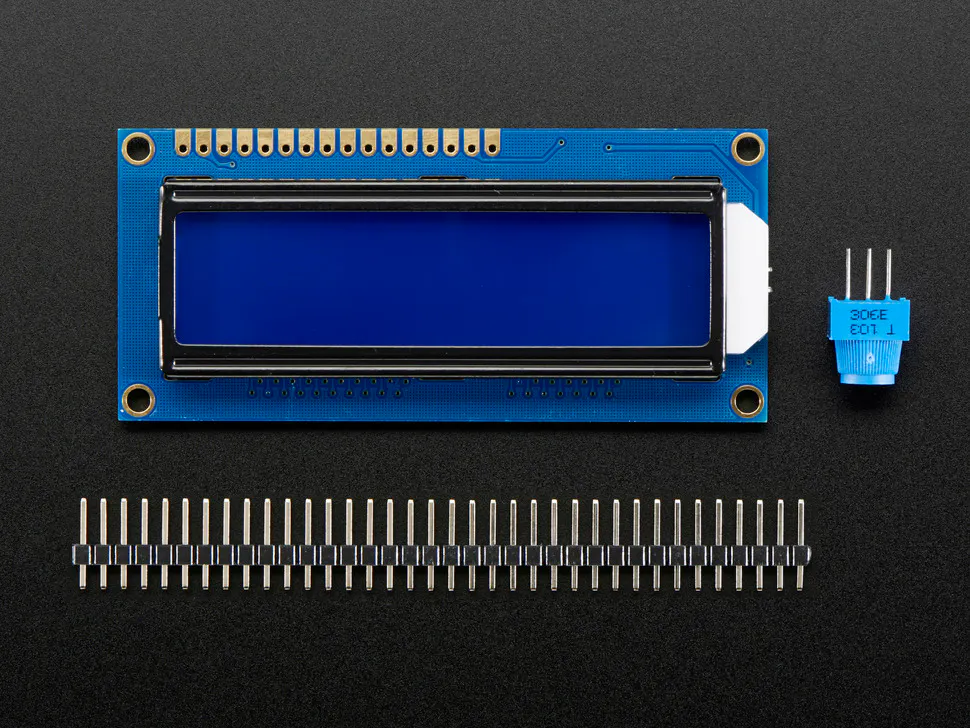Published

# Coin Counter Simulator

Demo program for using a 16x2 LCD display with a TI Launchpad

BeginnerShowcase (no instructions)30 minutes2,668## Things used in this project

### Hardware components×1Adafruit Standard LCD - 16x2 White on Blue
×1SparkFun Pushbutton switch 12mm
×4Jumper wires (generic)
×1Resistor 10k ohm
×4

### Software apps and online servicesTexas Instruments Energia

## Code

### Source Code

C/C++
```// include the library code:
#include <LiquidCrystal.h>

// initialize the library with the numbers of the interface pins
LiquidCrystal lcd(23, 24, 27,28, 29, 30, 34, 33, 32, 31);

const short buttonPenny= 19;
const short buttonNickel= 18;
const short buttonDime= 15;
const short buttonQuarter= 14;

short pennyButtonState = 0;
short nickelButtonState = 0;
short dimeButtonState = 0;
short quarterButtonState = 0;

unsigned int numPennies = 0;
unsigned int numNickels= 0;
unsigned int numDimes= 0;
unsigned int numQuarters = 0;
unsigned int totalDecimal=0;
unsigned int totalDollar=0;
unsigned int decimal=0;
int points= 0;

void setup() {
// set up the LCD's number of columns and rows:
lcd.begin (16, 2);
pinMode (buttonPenny, INPUT_PULLUP );
pinMode (buttonNickel, INPUT_PULLUP );
pinMode (buttonDime, INPUT_PULLUP );
pinMode (buttonQuarter, INPUT_PULLUP );
}

void loop() {

// set the cursor to column 0, line 1
// (note: line 1 is the second row, since counting begins with 0):
lcd.setCursor (0, 0);

if (pennyButtonState == 1){
lcd.clear();
numPennies++;
lcd.print("Penny");
delay (200);
}

if (nickelButtonState == 1){
lcd.clear();
numNickels++;
lcd.print("Nickel");
delay (200);
}

if (dimeButtonState == 1){
lcd.clear();
numDimes++;
lcd.print("Dime");
delay (200);
}

if (quarterButtonState == 1){
numQuarters++;
lcd.print("Quarter");
delay (200);
}

totalDecimal = numPennies+(numNickels*5)+(numDimes*10)+(numQuarters*25);
totalDollar = totalDecimal/100;
decimal = totalDecimal-100*totalDollar;

points=numPennies - (numNickels*5) - (numDimes*10) - (numQuarters*25);

lcd.setCursor (0,1);
lcd.print ("\$");

lcd.print(totalDollar );
lcd.print(".");

if(decimal < 10)
{
lcd.print("0");
}

lcd.print(decimal);

lcd.setCursor (8,0);
lcd.print(points);
lcd.print(" pts");

}
```

## Credits

### Ali Soomar

11 projects • 37 followers
Student at the University of Texas at Austin